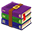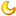# 电话拨号按键LCD显示模拟 51单片机程序+Proteus仿真10 |0 | 2020-2-1 15:57:03 | 显示全部楼层 |阅读模式
 1．实验要求： 设计一个单片机监控的电话拨号键盘，将电话键盘中拨出的某一电话号码，显示在LCD显示屏上。电话键盘共有12个键，除了“0”～ “9”10个数字键外，还有“*”键用于实现退格功能，即清除输入的号码；“#”键用于清除显示屏上所有的数字显示。还要求每按下一个键要发出声响，以表示按下该键。 2．仿真实现说明：      本实验在Proteus下按设计要求用P1口扩展了12个键盘，其中每个键盘所代表的含义已在Proteus下用文本注出。在LCD显示中，第一行为设计者名，第二行开始显示所拨的电话号码，最多为16位（因为LCD第二行功能显示16个字符）。电话拨盘模拟c语言程序 仿真文件.zip (58.61 KB, 售价: 3 工控币) 2020-2-1 15:56 上传 点击文件名下载附件单片机源程序如下: #include #define uint unsigned int #define uchar unsigned char uchar keycode,DDram_value=0xc0; sbit rs=P2^0; sbit rw=P2^1; sbit e =P2^2; sbit speaker=P2^3; uchar code table[]={0x30,0x31,0x32,0x33,0x34,                     0x35,0x36,0x37,0x38,0x39,0x20}; uchar code table_designer[]=" HIT ZYG Design"; void lcd_delay(); void delay(uint n); void lcd_init(void); void lcd_busy(void); void lcd_wr_con(uchar c); void lcd_wr_data(uchar d); uchar checkkey(void); uchar keyscan(void); void main() {     uchar num;         lcd_init();         lcd_wr_con(0x80);         for(num=0;num<=14;num++)         {           lcd_wr_data(table_designer[num]);         }         while(1)         {            keycode=keyscan();            if((keycode>=0)&&(keycode<=9))            {               lcd_wr_con(0x06);                   lcd_wr_con(DDram_value);               lcd_wr_data(table[keycode]);                   DDram_value++;            }            else if(keycode==0x0a)            {                      lcd_wr_con(0x04);                   DDram_value--;                   if(DDram_value<=0xc0)                   {                            DDram_value=0xc0;                   }                   else if(DDram_value>=0xcf)                   {                            DDram_value=0xcf;                   }                   lcd_wr_con(DDram_value);                   lcd_wr_data(table);            }            else if(keycode==0x0b)            {                      uchar i,j;                   j=0xc0;                   for(i=0;i<=15;i++)                   {                     lcd_wr_con(j);                           lcd_wr_data(table);                         j++;                   }                   DDram_value=0xc0;            }         } } //液晶工作时的延时函数 void lcd_delay() {   uchar y;   for(y=0;y<0xff;y++)   {            ;   } } //液晶初始化 void lcd_init(void) {    lcd_wr_con(0x01);    lcd_wr_con(0x38);    lcd_wr_con(0x0c);    lcd_wr_con(0x06); } // 判断液晶忙或闲的程序 void lcd_busy(void) {   P0=0xff;   rs=0;   rw=1;   e=1;   e=0;   while(P0&0x80)   {            e=0;          e=1;   }   lcd_delay(); } //向液晶控制口输入命令的调用函数 void lcd_wr_con(uchar c) {    lcd_busy();    e=0;    rs=0;    rw=0;    e=1;    P0=c;    e=0;    lcd_delay(); } //向液晶写数据的调用函数 void lcd_wr_data(uchar d) {    lcd_busy();    e=0;    rs=1;    rw=0;    e=1;    P0=d;    e=0;    lcd_delay(); } void delay(uint n) {    uchar i;    uint j;    for(i=50;i>0;i--)      for(j=n;j>0;j--); } //检测键有无按下函数 uchar checkkey(void) {         uchar temp;         P1=0xf0;         temp=P1;         temp=temp&0xf0;         if(temp==0xf0)         {            return(0);         }         else         {            return(1);         } } //键盘扫描函数，返回所按下的键盘号 uchar keyscan(void) {    uchar hanghao,liehao,keyvalue,buff;    if(checkkey()==0)    {              return(0xff);//无键按下，返回0xff    }    else    {              uchar sound;                  for(sound=50;sound>0;sound--)                  {                          speaker=0;                         delay(1);                         speaker=1;                         delay(1);                  }                  P1=0x0f;                  buff=P1;                  if(buff==0x0e)                  {                       hanghao=0;                  }                  else if(buff==0x0d)                  {                       hanghao=3;                  }                  else if(buff==0x0b)                  {                       hanghao=6;                  }                  else if(buff==0x07)                  {                       hanghao=9;                  }                  P1=0xf0;                  buff=P1;                  if(buff==0xe0)                  {                    liehao=2;                  }                  else if(buff==0xd0)                  {                          liehao=1;                  }                  else if(buff==0xb0)                  {                     liehao=0;                  }                  keyvalue=hanghao+liehao;                  while(P1!=0xf0);                  return(keyvalue);    } }复制代码

 本版积分规则 回帖后跳转到最后一页426主题 366积分366